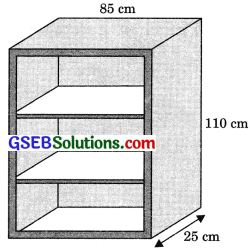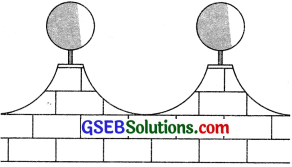# GSEB Solutions Class 9 Maths Chapter 13 Surface Areas and Volumes Ex 13.9

Gujarat Board GSEB Solutions Class 9 Maths Chapter 13 Surface Areas and Volumes Ex 13.9 Textbook Questions and Answers.

## Gujarat Board Textbook Solutions Class 9 Maths Chapter 13 Surface Areas and Volumes Ex 13.9

Question 1.
A wooden bookshelf has external dimensions as follows:
Height = 110 cm,
Depth = 25 cm,
Breadth = 85 cm (See figure).The thickness of the plank is 5 cm everywhere. The external faces are to be polished and the inner faces are to be painted. If the rate of polishing is 20 paise per cm2 and the rate of painting is 10 paise per cm2, find the total expenses required for polishing and painting the surface of the bookshelf.
Solution:
Surface area to be polished
= [(110 x 85) + 2(110 x 25) + 2(85 x 25) + 2(110 X 5) + 4(75 x 5)1
= (9350 + 5500 + 4250 + 1100 + 1500) cm2 = 21700 cm2
∴ Expenses required for polishing @ 20 paise per cm2
= 21700 x 20 paise
= ₹ $$\frac{21700 \times 20}{100}$$ = ₹ 4340Surface area to be painted
= [2(20 x 90) + 6(75 x 20) + (75 x 90)1
= (3600 + 9000 + 6750) cm2 = 19350 cm2
∴ Expenses required for painting @ 10 paise per cm2
= 19350 x lo paise
= ₹ $$\frac{19350 \times 10}{100}$$ = ₹ 1935
∴ Total expenses required for polishing and painting the surface of the bookshelf
= ₹4340 + ₹1935 = ₹6275

Question 2.
The front compound wall of a house is decorated by wooden spheres of diameter 21 cm, placed on small supports as shown in figure. Eight such spheres are used for this purpose and are to be painted silver. Each support is a cylinder of radius 1.5 cm and height 7 cm and is to be painted black. Find the cost of paint required if silver paint costs 25 paise per cm2 and black paint costs 5 paise per cm2.Solution:
For a wooden sphere
Diameter = 21 cm
∴ Radius (r) = $$\frac {21}{2}$$ cm
∴ Surface area of a wooden sphere
= 4πr2 = 4 x $$\frac {22}{7}$$ x ($$\frac {21}{2}$$)2
= 1386 cm2
∴ Surface area of a wooden sphere to be painted
= 1386 – π(1.5)2
= 1386 – $$\frac {22}{7}$$ (1.5)2 = 1378.93 cm2
∴ Surface area of eight wooden spheres
= 1378.93 x 8 = 11031.44 cm2
∴ Cost of painting silver @ 25 paise per cm2
= 11031.44 x 25 paise
= ₹ $$\frac{11031.44 \times 25}{100}$$
= ₹ 2757.86
For a cylindrical support
Height (h) = 7 cm∴ Surface area of a cylindrical support = 2πrh
= 2 x $$\frac {22}{7}$$ x 1.5 x 7 = 66 cm2
∴ Surface area of eight cylindrical supports
= 66 x 8 = 528 cm2
∴ Cost of painting black @ 25 paise per cm2
= 528 x 5 paise
= ₹ $$\frac{528 \times 5}{100}$$ = ₹ 26.40
∴ Cost of paint required
= (2757.86 + 26.40)
= 2784.26 (approx.)

Question 3
The diameter of a sphere is decreased by 25%. By what percent does its curved surface area decrease?
Solution:
Let the radius of the sphere be $$\frac {r}{2}$$cm.
Then its diameter = 2 $$\frac {r}{2}$$ = r cm
Curved surface area of the original sphere
4π($$\frac {r}{2}$$)2 = πr2 cm2
New diameter (decreased) of the sphere
= r – r x $$\frac {25}{100}$$
= r – $$\frac {r}{4}$$ = $$\frac {3r}{4}$$ cm
∴ Radius of the new sphere
= $$\frac {1}{2}$$($$\frac {3r}{4}$$) = $$\frac {3r}{8}$$cm
∴ New curved surface area of the sphere
4π($$\frac {3r}{8}$$)2 = $$\frac{9 \pi r^{2}}{16}$$ cm2
∴ Decrease in the original curved surfàce area
= πr2 – $$\frac{9 \pi r^{2}}{16}$$
= $$\frac{16 \pi r^{2}-9 \pi r^{2}}{16}$$ = $$\frac{7 \pi r^{2}}{16}$$
∴ Percentage of decrease in the original curved surface area
= $$\frac{\frac{7 \pi r^{2}}{16}}{\pi r^{2}} \times 100 \%$$
= $$\frac{700}{16} \%$$ = $$\frac{175}{4} \%$$
= $$43 \frac{3}{4} \%$$ or 43.75%
Hence, the original curved surface area decreases by 43.75%.